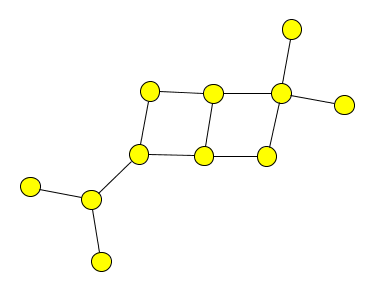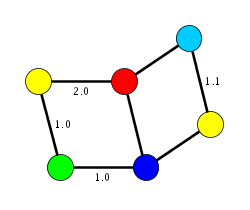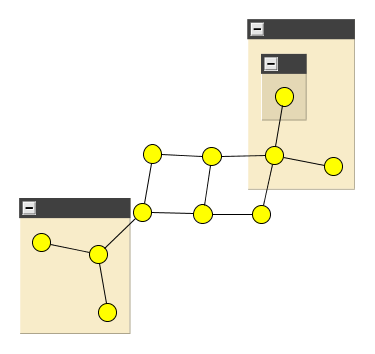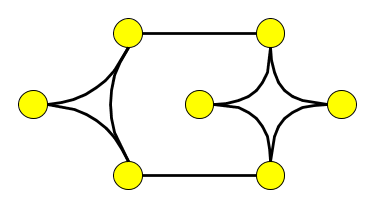# GraphML Primer

Editors:
Ulrik Brandes ulrik.brandes@uni-konstanz.de,
Markus Eiglsperger eiglsper@web.de,
Jürgen Lerner lerner@inf.uni-konstanz.de

## Abstract

GraphML Primer is a non-normative document intended to provide an easily readable description of the GraphML facilities, and is oriented towards quickly understanding how to create GraphML documents. This primer describes the language features through examples which are complemented by references to normative texts.

1 Introduction
2 Basic Concepts
2.1 A Simple Graph
2.3 Graph Tolology
2.3.1 Declaring a Graph
2.3.2 Declaring a Node
2.3.3 Declaring an Edge
2.4 Attributes
2.4.1 GraphML-Attributes Example
2.4.2 Declaring GraphML-Attributes
2.4.4 Defining GraphML-Attribute Values
2.5 Parse Info
3 Advanced Concepts I: Nested Graphs, Hyperedges & Ports
3.1 Nested Graphs
3.2 Hyperedges
3.3 Ports
4 Advanced Concepts II: Extending GraphML
4.1 Adding XML Attributes to GraphML Elements

## 1 Introduction

This document, GraphML Primer, provides an description of GraphML, and should be used alongside the formal descriptions of the language contained in the GraphML specification. The intended audience of this document includes application developers whose programs read and write GraphML files, and users who want to communicate with programs using GraphML import/export. The text assumes that you have a basic understanding of XML 1.0 and XML-Namespaces. Basic knowledge of XML Schema is also assumed for some parts of this document. Each major section of the primer introduces new features of the language, and describes those features in the context of concrete examples.

Section 2 covers the basic mechanisms of GraphML. It describes how to declare a simple graph by defining its nodes and edges and how to add simple user data to the graph.

Section 3 describes advanced graph models which include nested graphs, hyperedges, and ports.

Section 4 describes mechanisms for extending GraphML to store complex application specific data.

The primer is a non-normative document, which means that it does not provide a definitive specification of the GraphML language. The examples and other explanatory material in this document are provided to help you understand GraphML, but they may not always provide definitive answers. In such cases, you will need to refer to the GraphML specification, and to help you do this, we provide many links pointing to the relevant parts of the specification.

## 2 Basic Concepts

The purpose of a GraphML document is to define a graph. Let us start by considering the graph shown in the figure below. It contains 11 nodes and 12 edges.

A simple graph### 2.1 A Simple Graph

The graph is contained in the file ``` simple.graphml```:

A simple graph
```<?xml version="1.0" encoding="UTF-8"?>
<graphml xmlns="http://graphml.graphdrawing.org/xmlns"
xmlns:xsi="http://www.w3.org/2001/XMLSchema-instance"
xsi:schemaLocation="http://graphml.graphdrawing.org/xmlns
http://graphml.graphdrawing.org/xmlns/1.0/graphml.xsd">
<graph id="G" edgedefault="undirected">
<node id="n0"/>
<node id="n1"/>
<node id="n2"/>
<node id="n3"/>
<node id="n4"/>
<node id="n5"/>
<node id="n6"/>
<node id="n7"/>
<node id="n8"/>
<node id="n9"/>
<node id="n10"/>
<edge source="n0" target="n2"/>
<edge source="n1" target="n2"/>
<edge source="n2" target="n3"/>
<edge source="n3" target="n5"/>
<edge source="n3" target="n4"/>
<edge source="n4" target="n6"/>
<edge source="n6" target="n5"/>
<edge source="n5" target="n7"/>
<edge source="n6" target="n8"/>
<edge source="n8" target="n7"/>
<edge source="n8" target="n9"/>
<edge source="n8" target="n10"/>
</graph>
</graphml>
```

The GraphML document consists of a `graphml` element and a variety of subelements: `graph`, `node`, `edge`. In the remainder of this section we will discuss these elements in detail and show how they define a graph.

In this section we discuss the parts of the document which are common to all GraphML documents, basically the `graphml` element.

A Header with XML Schema reference
```<?xml version="1.0" encoding="UTF-8"?>
<graphml xmlns="http://graphml.graphdrawing.org/xmlns"
xmlns:xsi="http://www.w3.org/2001/XMLSchema-instance"
xsi:schemaLocation="http://graphml.graphdrawing.org/xmlns
http://graphml.graphdrawing.org/xmlns/1.0/graphml.xsd">

...

</graphml>
```

The first line of the document is an XML process instruction which defines that the document adheres to the XML 1.0 standard and that the encoding of the document is UTF-8, the standard encoding for XML documents. Of course other encodings can be chosen for GraphML documents.

The second line contains the root-element element of a GraphML document: the `graphml` element. The `graphml` element, like all other GraphML elements, belongs to the namespace `http://graphml.graphdrawing.org/xmlns`. For this reason we define this namespace as the default namespace in the document by adding the XML Attribute `xmlns="http://graphml.graphdrawing.org/xmlns"` to it. The two other XML Attributes are needed to specify the XML Schema for this document. In our example we use the standard schema for GraphML documents located on the `graphdrawing.org` server. The first attribute, `xmlns:xsi="http://www.w3.org/2001/XMLSchema-instance"`, defines `xsi` as the XML Schema namespace. The second attribute, ```xsi:schemaLocation="http://graphml.graphdrawing.org/xmlns http://graphml.graphdrawing.org/xmlns/1.0/graphml.xsd" ```, defines the XML Schema location for all elements in the GraphML namespace.

The XML Schema reference is not required but it provides means to validate the document and is therefore strongly recommended.

A Header without XML Schema reference
```<?xml version="1.0" encoding="UTF-8"?>
<graphml xmlns="http://graphml.graphdrawing.org/xmlns">

...

</graphml>
```

### 2.3 The Graph

A graph is, not surprisingly, denoted by a `graph` element. Nested inside a `graph` element are the declarations of nodes and edges. A node is declared with a `node` element, and an egde with an `edge` element.

```  <graph id="G" edgedefault="directed">
<node id="n0"/>
<node id="n1"/>
...
<node id="n10"/>
<edge source="n0" target="n2"/>
<edge source="n1" target="n2"/>
...
<edge source="n8" target="n10"/>
</graph>
```

In GraphML there is no order defined for the appearance of `node` and `edge` elements. Therefore the following example is a perfectly valid GraphML fragment:

```  <graph id="G" edgedefault="directed">
<node id="n0"/>
<edge source="n0" target="n2"/>
<node id="n1"/>
<node id="n2"/>
...
</graph>
```

#### 2.3.1 Declaring a Graph

Graphs in GraphML are mixed, in other words, they can contain directed and undirected edges at the same time. If no direction is specified when an edge is declared, the default direction is applied to the edge. The default direction is declared as the XML Attribute `edgedefault` of the `graph` element. The two possible value for this XML Attribute are `directed` and `undirected`. Note that the default direction must be specified.

Optionally an identifier for the graph can be specified with the XML Attribute `id`. The identifier is used, when it is necessary to reference the graph.

#### 2.3.2 Declaring a Node

Nodes in the graph are declared by the `node` element. Each node has an identifier, which must be unique within the entire document, i.e., in a document there must be no two nodes with the same identifier. The identifier of a node is defined by the XML-Attribute `id`.

#### 2.3.3 Declaring an Edge

Edges in the graph are declared by the `edge` element. Each edge must define its two endpoints with the XML-Attributes `source` and `target`. The value of the `source`, resp. `target`, must be the identifier of a node in the same document.

Edges with only one endpoint, also called loops, selfloops, or reflexive edges, are defined by having the same value for `source` and `target`.

The optional XML-Attribute `directed` declares if the edge is directed or undirected. The value `true` declares a directed edge, the value `false` an undirected edge. If the direction is not explicitely defined, the default direction is applied to this edge as defined in the enclosing graph.

Optionally an identifier for the edge can be specified with the XML Attribute `id`. When it is necessary to reference the edge, the `id` XML-Attribute is used.

```
...
<edge id="e1" directed="true" source="n0" target="n2"/>
...
```

## 2.4 GraphML-Attributes

In the previous section we discussed how to describe the topology of a graph in GraphML. While pure topological information may be sufficient for some appications of GraphML, for the most time additional information is needed. With the help of the extension GraphML-Attributes one can specify additional information of simple type for the elements of the graph. Simple type means that the information is restricted to scalar values, e.g. numerical values and strings.

If you want to add structured content to graph elements you should use the key/data extension mechanism of GraphML. For a detailed description of this mechanism see Chapter 4. GraphML-Attributes themselfes are specialized data/key extensions.

GraphML-Attributes must not be confounded with XML-Attributes which are a different concept.

### 2.4.1 GraphML-Attributes Example

In this section a graph with colored nodes and edge weights will be our running example.

A graph with colored nodes and edge weights.We will use GraphML-Attributes to store the extra data on the nodes and edges. The file attributes.graphml shows the result:

```<?xml version="1.0" encoding="UTF-8"?>
<graphml xmlns="http://graphml.graphdrawing.org/xmlns"
xmlns:xsi="http://www.w3.org/2001/XMLSchema-instance"
xsi:schemaLocation="http://graphml.graphdrawing.org/xmlns
http://graphml.graphdrawing.org/xmlns/1.0/graphml.xsd">
<key id="d0" for="node" attr.name="color" attr.type="string">
<default>yellow</default>
</key>
<key id="d1" for="edge" attr.name="weight" attr.type="double"/>
<graph id="G" edgedefault="undirected">
<node id="n0">
<data key="d0">green</data>
</node>
<node id="n1"/>
<node id="n2">
<data key="d0">blue</data>
</node>
<node id="n3">
<data key="d0">red</data>
</node>
<node id="n4"/>
<node id="n5">
<data key="d0">turquoise</data>
</node>
<edge id="e0" source="n0" target="n2">
<data key="d1">1.0</data>
</edge>
<edge id="e1" source="n0" target="n1">
<data key="d1">1.0</data>
</edge>
<edge id="e2" source="n1" target="n3">
<data key="d1">2.0</data>
</edge>
<edge id="e3" source="n3" target="n2"/>
<edge id="e4" source="n2" target="n4"/>
<edge id="e5" source="n3" target="n5"/>
<edge id="e6" source="n5" target="n4">
<data key="d1">1.1</data>
</edge>
</graph>
</graphml>
```

### 2.4.2 Declaring GraphML-Attributes

A GraphML-Attribute is defined by a `key` element which specifies the identifier, name, type and domain of the attribute.

The identifier is specified by the XML-Attribute `id` and is used to refer to the GraphML-Attribute inside the document.

The name of the GraphML-Attribute is defined by the XML-Attribute `attr.name` and must be unique among all GraphML-Attributes declared in the document. The purpose of the name is that applications can identify the meaning of the attribute. Note that the name of the GraphML-Attribute is not used inside the document, the identifier is used for this purpose.

The type of the GraphML-Attribute can be either `boolean`, `int`, `long`, `float`, `double`, or `string`. These types are defined like the corresponding types in the Java(TM)-Programming language.

The domain of the GraphML-Attribute specifies for which graph elements the GraphML-Attribute is declared. Possible values include `graph`, `node`, `edge`, and `all`.

```
...
<key id="d1" for="edge" attr.name="weight" attr.type="double"/>
...
```

It is possible to define a default value for a GraphML-Attribute. The text content of the `default` element defines this default value.

```
...
<key id="d0" for="node" attr.name="color" attr.type="string">
<default>yellow</default>
</key>
...
```

### 2.4.3 Defining GraphML-Attribute Values

The value of a GraphML-Attribute for a graph element is defined by a `data` element nested inside the element for the graph element. The `data` element has an XML-Attribute `key`, which refers to the identifier of the GraphML-Attribute. The value of the GraphML-Attribute is the text content of the `data` element. This value must be of the type declared in the corresponding `key` definition.

```  ...
<key id="d0" for="node" attr.name="color" attr.type="string">
<default>yellow</default>
</key>
<key id="d1" for="edge" attr.name="weight" attr.type="double"/>
<graph id="G" edgedefault="undirected">
<node id="n0">
<data key="d0">green</data>
</node>
<node id="n1"/>
...
<edge id="e0" source="n0" target="n2">
<data key="d1">1.0</data>
</edge>
<edge id="e1" source="n0" target="n1">
<data key="d1">1.0</data>
</edge>
<edge id="e2" source="n1" target="n3">
<data key="d1">2.0</data>
</edge>
<edge id="e3" source="n3" target="n2"/>
...
</graph>
...
```

There can be graph elements for which a GraphML-Attribute is defined but no value is declared by a corresponding `data` element. If a default value is defined for this GraphML-Attribute, then this default value is applied to the graph element. In the above example no value is defined for the node with identifier `n1` and the GraphML-Attribute with name `color`. Therefore this GraphML-Attribute has the default value, `yellow` for this node. If no default value is specified, as for the GraphML-Attribute `weight` in the above example, the value of the GraphML-Attribute is undefined for the graph element. In the above example the value is undefined of the GraphML-Attribute weight for the edge with identifier `e3`.

## 2.5 Parse Info

To make it possible to implement optimized parsers for GraphML documents meta-data can be attached as XML-Attributes to some GraphML elements. All XML-Attributes denoting meta-data are prefixed with `parse`. There are two kinds of meta-data: information about the number of elements and information how specific data is encoded in the document.

For the first kind, information about the number of elements, the following XML-Attributes for the `graph` element are defined: The XML-Attribute `parse.nodes` denotes the number of nodes in the graph, the XML-Attribute `parse.edges`the number of edges. The XML-Attribute `parse.maxindegree` denotes the maximum indegree of the nodes in the graph and the XML-Attribute `parse.maxoutdegree` the maximum outdegree. For the `node` element the XML-Attribute `parse.indegree` denotes the indegree of the node and the XML-Attribute `parse.outdegree` the outdegree.

For the second kind, information about element encoding, the following XML-Attributes for the `graph` element are defined: If the XML-Attribute `parse.nodeids` has the value `canonical`, all nodes have identifiers following the pattern `nX`, where `X` denotes the number of occurences of the `node` element before the current element. Otherwise the value of the XML-Attribute is `free`. The same holds for edges for which the corresponding XML-Attribute `parse.edgeids` is defined, with the only difference that the identifiers of the edges follow the pattern `eX`. The XML-Attribute `parse.order` denotes the order in which `node` and `edge` elements occur in the document. For the value `nodesfirst` no `node` element is allowed to occur after the first occurence of an `edge` element. For the value `adjacencylist`, the declaration of a node is followed by the declaration of its adjacent edges. For the value `free` no order is imposed.

The following example demonstrates the parse info meta-data on our running example:

A graph with additional parse info attributes.
```<?xml version="1.0" encoding="UTF-8"?>
<!-- This file was written by the JAVA GraphML Library.-->
<graphml xmlns="http://graphml.graphdrawing.org/xmlns"
xmlns:xsi="http://www.w3.org/2001/XMLSchema-instance"
xsi:schemaLocation="http://graphml.graphdrawing.org/xmlns
http://graphml.graphdrawing.org/xmlns/1.0/graphml.xsd">
<graph id="G" edgedefault="directed"
parse.nodes="11" parse.edges="12"
parse.maxindegree="2" parse.maxoutdegree="3"
parse.nodeids="canonical" parse.edgeids="free"
parse.order="nodesfirst">
<node id="n0" parse.indegree="0" parse.outdegree="1"/>
<node id="n1" parse.indegree="0" parse.outdegree="1"/>
<node id="n2" parse.indegree="2" parse.outdegree="1"/>
<node id="n3" parse.indegree="1" parse.outdegree="2"/>
<node id="n4" parse.indegree="1" parse.outdegree="1"/>
<node id="n5" parse.indegree="2" parse.outdegree="1"/>
<node id="n6" parse.indegree="1" parse.outdegree="2"/>
<node id="n7" parse.indegree="2" parse.outdegree="0"/>
<node id="n8" parse.indegree="1" parse.outdegree="3"/>
<node id="n9" parse.indegree="1" parse.outdegree="0"/>
<node id="n10" parse.indegree="1" parse.outdegree="0"/>
<edge id="edge0001" source="n0" target="n2"/>
<edge id="edge0002" source="n1" target="n2"/>
<edge id="edge0003" source="n2" target="n3"/>
<edge id="edge0004" source="n3" target="n5"/>
<edge id="edge0005" source="n3" target="n4"/>
<edge id="edge0006" source="n4" target="n6"/>
<edge id="edge0007" source="n6" target="n5"/>
<edge id="edge0008" source="n5" target="n7"/>
<edge id="edge0009" source="n6" target="n8"/>
<edge id="edge0010" source="n8" target="n7"/>
<edge id="edge0011" source="n8" target="n9"/>
<edge id="edge0012" source="n8" target="n10"/>
</graph>
</graphml>
```

## 3. Advanced Concepts I: Nested Graphs, Hyperedges & Ports

In some applications the graph model described in the previous section is too restrictive and does not model adequatly the application data.

In this section we discuss advanced graph models which can model a nesting hierarchy, hyperedges and ports.

### 3.1 Nested Graphs

GraphML supports nested graphs, i.e., graphs in which the nodes are hierarchically ordered. The hierarchy is expressed by the structure of the GraphML document. A node in a GraphML document may have a `graph` element which itself contains the nodes which are in the hierarchy below this node. Here is an example for a nested graph and the corresponding GraphML document. Note that in the drawing of the graph the hierarchy is expressed by containment, i.e., the a node a is below a node b in the hierarchy if and only if the graphical representation of a is entirely inside the graphical representation of b.

A nested graph.The file nested.graphml shows the corresponding GraphML document:

```<?xml version="1.0" encoding="UTF-8"?>
<graphml xmlns="http://graphml.graphdrawing.org/xmlns"  xmlns:xsi="http://www.w3.org/2001/XMLSchema-instance"
xsi:schemaLocation="http://graphml.graphdrawing.org/xmlns http://graphml.graphdrawing.org/xmlns/1.0/graphml.xsd">
<graph id="G" edgedefault="undirected">
<node id="n0"/>
<node id="n1"/>
<node id="n2"/>
<node id="n3"/>
<node id="n4"/>
<node id="n5">
<graph id="n5:" edgedefault="undirected">
<node id="n5::n0"/>
<node id="n5::n1"/>
<node id="n5::n2"/>
<edge id="e0" source="n5::n0" target="n5::n2"/>
<edge id="e1" source="n5::n1" target="n5::n2"/>
</graph>
</node>
<node id="n6">
<graph id="n6:" edgedefault="undirected">
<node id="n6::n0">
<graph id="n6::n0:" edgedefault="undirected">
<node id="n6::n0::n0"/>
</graph>
</node>
<node id="n6::n1"/>
<node id="n6::n2"/>
<edge id="e10" source="n6::n1" target="n6::n0::n0"/>
<edge id="e11" source="n6::n1" target="n6::n2"/>
</graph>
</node>
<edge id="e2" source="n5::n2" target="n0"/>
<edge id="e3" source="n0" target="n2"/>
<edge id="e4" source="n0" target="n1"/>
<edge id="e5" source="n1" target="n3"/>
<edge id="e6" source="n3" target="n2"/>
<edge id="e7" source="n2" target="n4"/>
<edge id="e8" source="n3" target="n6::n1"/>
<edge id="e9" source="n6::n1" target="n4"/>
</graph>
</graphml>
```

The edges between two nodes in a nested graph have to be declared in a graph, which is an ancestor of both nodes in the hierarchy. Note that this is true for our example. Declaring the edge between node `n6::n1` and node `n4::n0::n0` inside graph `n6::n0` would be wrong while declaring it in graph `G` would be correct. A good policy is to place the edges at the least common ancestor of the nodes in the hierarchy, or at the top level.

For applications which can not handle nested graphs the fall-back behaviour is to ignore nodes which are not contained in the top-level graph and to ignore edges which have do not have both endpoints in the top-level graph.

### 3.2 Hyperedges

Hyperedges are a generalization of edges in the sense that they do not only relate two endpoints to each other, they express a relation between an arbitrary number of enpoints. Hyperedges are declared by a `hyperedge` element in GraphML. For each enpoint of the hyperedge, this hyperedge element contains an `endpoint` element. The `endpoint` element must have an XML-Attribute `node`, which contains the identifier of a node in the document. The following example contains two hyperedges and two edges. The hyperedges are illustrated by joining arcs, the edges by straight lines. Note that edges can be either specified by an `edge` element or by a `hyperedge` element containing two `endpoint` elements.
A graph with hyperedges.The file hyper.graphml shows the corresponding GraphML document:

```<?xml version="1.0" encoding="UTF-8"?>
<graphml xmlns="http://graphml.graphdrawing.org/xmlns"  xmlns:xsi="http://www.w3.org/2001/XMLSchema-instance"
xsi:schemaLocation="http://graphml.graphdrawing.org/xmlns http://graphml.graphdrawing.org/xmlns/1.0/graphml.xsd">
<graph id="G" edgedefault="undirected">
<node id="n0"/>
<node id="n1"/>
<node id="n2"/>
<node id="n3"/>
<node id="n4"/>
<node id="n5"/>
<node id="n6"/>
<hyperedge>
<endpoint node="n0"/>
<endpoint node="n1"/>
<endpoint node="n2"/>
</hyperedge>
<hyperedge>
<endpoint node="n3"/>
<endpoint node="n4"/>
<endpoint node="n5"/>
<endpoint node="n6"/>
</hyperedge>
<hyperedge>
<endpoint node="n1"/>
<endpoint node="n3"/>
</hyperedge>
<edge source="n0" target="n4"/>
</graph>
</graphml>
```

Like edges, hyperedges and enpoints may have an XML-Attribute `id`, which defines a unique identifier for the corresponding element.

### 3.3 Ports

A node may specify different logical locations for edges and hyperedges to connect. The logical locations are called "ports". As an analogy, think of the graph as a motherboard, the nodes as integrated circuits and the edges as connecting wires. Then the pins on the integrated circuits correspond to ports of a node.

The ports of a node are declared by `port` elements as children of the corresponding `node` elements. Note that port elements may be nested, i.e., they may contain `port` elements themselves. Each `port` element must have an XML-Attribute `name`, which is an identifier for this port. The `edge` element has optional XML-Attributes `sourceport` and `targetport` with which an edge may specify the port on the source, resp. target, node. Correspondingly, the `endpoint` element has an optional XML-Attribute `port`.

The document port.graphml is an example for a document with ports:

```<?xml version="1.0" encoding="UTF-8"?>
<graphml xmlns="http://graphml.graphdrawing.org/xmlns"  xmlns:xsi="http://www.w3.org/2001/XMLSchema-instance"
xsi:schemaLocation="http://graphml.graphdrawing.org/xmlns http://graphml.graphdrawing.org/xmlns/1.0/graphml.xsd">
<graph id="G" edgedefault="directed">
<node id="n0">
<port name="North"/>
<port name="South"/>
<port name="East"/>
<port name="West"/>
</node>
<node id="n1">
<port name="North"/>
<port name="South"/>
<port name="East"/>
<port name="West"/>
</node>
<node id="n2">
<port name="NorthWest"/>
<port name="SouthEast"/>
</node>
<node id="n3">
<port name="NorthEast"/>
<port name="SouthWest"/>
</node>
<edge source="n0" target="n3" sourceport="North" targetport="NorthEast"/>
<hyperedge>
<endpoint node="n0" port="North"/>
<endpoint node="n1" port="East"/>
<endpoint node="n2" port="SouthEast"/>
</hyperedge>
</graph>
</graphml>
```

# 4. Advanced Concepts II: Extending GraphML

GraphML is designed to be easily extensible. With GraphML the topology of a graph and simple attributes of graph elements can be serialized. To store more complex application data one has to extend GraphML. In this section we will discuss the different possibilities to extend GraphML.

Extensions of GraphML should be defined by an XML Schema. The Schema which defines the extension can be derived from the GraphML Schema documents by using a standard mechanism similar to the one used by XHTML.

### 4.1 Adding XML Attributes to GraphML Elements

In most cases, additional information can (and should) be attached to GraphML elements by usage of GraphML-Attributes. This assures readability for other GraphML parsers. However, sometimes it might be more convenient to use XML attributes. Suppose you have a parser which knows the XLink `href` attribute and interprets it correctly as a URL. Suppose further you want to store a graph, whose nodes model WWW pages, in GraphML. To associate a node to the page it models you write the page's URL in an `xlink:href` attribute within the `node`-tag:

```    ...
...
```

To add XML attributes to GraphML elements one has to extend GraphML. This extension can be defined by an XML Schema. The document graphml+xlink.xsd shows how the `href` attribute is added to `node`. (Note that, until now, there is no official Schema definition for XLink; to validate the following file, make sure that the attribute `schemaLocation` points to some preliminary version, e.g. `http://graphml.graphdrawing.org/xmlns/1.0/xlink.xsd`.)

```<?xml version="1.0" encoding="UTF-8"?>
<xs:schema
targetNamespace="http://graphml.graphdrawing.org/xmlns"
xmlns="http://graphml.graphdrawing.org/xmlns"
xmlns:xs="http://www.w3.org/2001/XMLSchema"
elementFormDefault="qualified"
attributeFormDefault="unqualified"
>

<xs:redefine
schemaLocation="http://graphml.graphdrawing.org/xmlns/1.0/graphml.xsd">
<xs:attributeGroup name="node.extra.attrib">
<xs:attributeGroup ref="node.extra.attrib"/>
</xs:attributeGroup>
</xs:redefine>

</xs:schema>
```

The parts in the above document have the following function: The document graphml+xlink.xsd has a `schema` element as its root element. `targetNamespace="http://graphml.graphdrawing.org/xmlns"` says that the language defined by this document is GraphML. The next three lines specify the default namespace and the namespace prefixes for XLink and XMLSchema. The attributes `elementFormDefault` and `attributeFormDefault` are of no importance for this example.
```<xs:import namespace="http://www.w3.org/1999/xlink" schemaLocation="xlink.xsd"/>``` gives access to the XLink namespace, located at the file `xlink.xsd`.
```<xs:redefine schemaLocation="http://graphml.graphdrawing.org/xmlns/1.0/graphml.xsd"> ``` specifies the file, (part of) which is being redefined. The attribute group `node.extra.attrib` is included in the attribute-list of `node`. After redefinition, this attribute group has its old content plus one more attribute, namely `xlink:href`, which is optional.

Besides `node.extra.attrib`, there are corresponding attribute groups for all major GraphML elements.

The document attributes.ext.graphml is an example for a document which is valid with respect to the schema graphml+xlink.xsd.

```<?xml version="1.0" encoding="UTF-8"?>
<graphml xmlns="http://graphml.graphdrawing.org/xmlns"
xmlns:xsi="http://www.w3.org/2001/XMLSchema-instance"
xsi:schemaLocation="http://graphml.graphdrawing.org/xmlns
<graph edgedefault="directed">
<node id="n1" />
<edge source="n0" target="n1"/>
</graph>
</graphml>
```

Structured content can be added within the `data` element. For example a user wants to store images for nodes, written in SVG.

```    ...
xmlns:svg="http://www.w3.org/2000/svg"
...
<node id="n0" >
<data key="k0">
<svg:svg width="4cm" height="8cm" version="1.1">
<svg:ellipse cx="2cm" cy="4cm" rx="2cm" ry="1cm" />
</svg:svg>
</data>
</node>
...
```

To add structured data to GraphML elements one has to extend GraphML. This extension can be defined by an XML Schema. The document graphml+svg.xsd shows how SVG elements are added to the content of `data`. (Note that, until now, there is no official Schema definition for SVG; to validate the following file, make sure that the attribute `schemaLocation` points to some preliminary version, e.g. `http://www.w3.org/TR/2002/WD-SVG11-20020108/SVG.xsd`.)

```<?xml version="1.0" encoding="UTF-8"?>
<xs:schema
targetNamespace="http://graphml.graphdrawing.org/xmlns"
xmlns="http://graphml.graphdrawing.org/xmlns"
xmlns:svg="http://www.w3.org/2000/svg"
xmlns:xs="http://www.w3.org/2001/XMLSchema"
elementFormDefault="qualified"
attributeFormDefault="unqualified"
>

<xs:import namespace="http://www.w3.org/2000/svg"
schemaLocation="svg.xsd"/>

<xs:redefine
schemaLocation="http://graphml.graphdrawing.org/xmlns/1.0/graphml.xsd">
<xs:complexType name="data-extension.type">
<xs:complexContent>
<xs:extension base="data-extension.type">
<xs:sequence>
<xs:element ref="svg:svg"/>
</xs:sequence>
</xs:extension>
</xs:complexContent>
</xs:complexType>
</xs:redefine>

</xs:schema>
```

The above Schema is similar to the example in Adding Attributes: First the necessary namespace declarations are made. Then the SVG namespace is imported. Finally the complex type `data-extension.type`, which is the base-type for the content of the `data` element, is extended by the SVG element `svg`.

With the Schema in graphml+svg.xsd, the GraphML Document svg.graphml can be validated:

```<?xml version="1.0" encoding="UTF-8"?>
<graphml xmlns="http://graphml.graphdrawing.org/xmlns"
xmlns:svg="http://www.w3.org/2000/svg"
xmlns:xsi="http://www.w3.org/2001/XMLSchema-instance"
xsi:schemaLocation="http://graphml.graphdrawing.org/xmlns
graphml+svg.xsd">
<key id="k0" for="node">
<default>
<svg:svg width="5cm" height="4cm" version="1.1">
<svg:desc>Default graphical representation for nodes
</svg:desc>
<svg:rect x="0.5cm" y="0.5cm" width="2cm" height="1cm"/>
</svg:svg>
</default>
</key>
<key id="k1" for="edge">
<desc>Graphical representation for edges
</desc>
</key>
<graph edgedefault="directed">
<node id="n0">
<data key="k0">
<svg:svg width="4cm" height="8cm" version="1.1">
<svg:ellipse cx="2cm" cy="4cm" rx="2cm" ry="1cm" />
</svg:svg>
</data>
</node>
<node id="n1" />
<edge source="n0" target="n1">
<data key="k1">
<svg:svg width="12cm" height="4cm" viewBox="0 0 1200 400">
<svg:line x1="100" y1="300" x2="300" y2="100"
stroke-width="5"  />
</svg:svg>
</data>
</edge>
</graph>
</graphml>
```

Note that the node with id `n1` admits the default graphical representation given in `key k0`. The above example shows also the usefulness of XML Namespaces: there are two different `desc` elements - one in the GraphML namespace and one in the SVG namespace. Possible conflicts, due to elements from different XML languages that happen to have identical names, are resolved by different namespaces.